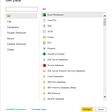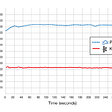# Developing the Intuition Behind Modern Portfolio Theory With an Interactive Visualization in Python

Anyone seeking to manage an investment portfolio has probably already been presented to the Modern Portfolio Theory (MPT), which was introduced in 1952 by Harry Markowitz in the work entitled “Portfolio Selection”, published by The Journal of Finance. The proposed framework is well documented and was extensively developed over the last decades, however some results are counter-intuitive and often taken for granted, making it easy to be overlooked by someone new in the field. In this article I will explore this results with an interactive visualization using Jupyter Widgets that will hopefully help you appreciate the beauty of the methodology. The purpose is not to make a comprehensive introduction to MPT and efficient frontier, but emphasize the power of combining risky assets in a portfolio.

## Risk and Return

Let's consider that we want to invest our money in stocks, and just to keep it simple, in this article we will consider investing in only two stocks, Stock A and Stock B. The question that follows is how do we split our money between these two assets? We can put 50% of our money into stock A and 50% into Stock B, but is that a good option?

At this point the theory proposed by Markowitz comes in place, which consists of a model to build an optimized portfolio of assets that maximizes the expected return for a given level of risk. So in order to apply it, we need to understand the return and risk characteristics of each asset, to finally combine them and assess the expected returns of our portfolio, as well as the risk we are willing to take to get this return.

Let’s look at how can we define these two characteristics of our stocks.

## Returns

Considering Pt the price of a security at time t, and Pt+1 the price of the security at time t+1, the return of this security from time t to time t+1 is given by:

This equation can be rearranged and expressed in a slightly more compact form:

These are called simple or raw returns. While in many cases log returns are preferable, for the purpose of this article, I will consider only simple returns.

## Risk

The most common parameter used to measure risk is the volatility, which is defined as the standard deviation of the returns, and is calculated dividing the squared deviations of the returns to the mean, then taking the square root.

## Building a Portfolio with 2 assets

Now that we have defined these two parameters, we can start to understand the relationship between assets A and B by plotting the returns on Y axis and volatility on X axis.

We are not interested at the moment in calculating these parameters, but in understanding how they contribute to portfolio construction. So, let’s assume that Stock A return Ra is 8%, and its volatility σ_a is equal to 12%. We can also assume that Stock B return Rb is 14%, and volatility σ_b is 16%. With those parameters, we can plot them using the following python code.

Considering now the case of a portfolio consisting of these two stocks, we can now assign weights to each of them, being wa the weight of Stock A and wb the weight of Stock B. In a simple case where we equally split our money into the two stocks (wa = wb = 50%), it is somehow intuitive to think that the return of the portfolio composed by them will be the weighted average of the returns:

We may be inclined to think that this is also the case of the volatility. However, the portfolio’s volatility is not simply given by the weighted average of each individual asset’s volatility, but depends also on the correlation between the assets. The expression for the portfolio volatility in the case of a two-assets portfolio is given by:

The term 𝜌 that appears in the above equation is the correlation of the two assets and can range from -1 (indicating a perfect inverse correlation) and 1 (perfect correlation). It is exactly this term that is responsible for the surprising results of the MPT. Only in the case of perfect correlated assets (𝜌=1) the volatility is simply the weighted average of the individual assets. In this specific case, the portfolio risk-return profile will look like a straight line between the individual assets, as shown below, and each point in the line represents different allocations or weights to each stock.

Nevertheless, in most practical cases the value of the correlation will not be equal to one, and will lie anywhere between -1 and 1. We can see what happens when we assign different values to the correlation and plot them on the above chart. In order to do that, we need to code the equations for portfolio return and portfolio volatility given before. We also need to assign varying weights for the stocks in a way that the sum of weights is always 1. This can be implemented with the following code:

Now, we can clearly see that by combining two assets that are not perfectly correlated (𝜌 different from 1), it is possible to build a portfolio with lower volatility than any of the individual assets. The lines shown above are known as the Efficient Frontier, and represent asset allocation that minimizes the risk of the portfolio for a given return.

In order to explore this characteristic even further, we can create an interactive visualization with Jupyter Widgets, allowing us to change all the parameters and instantly see their effects on the risk-return profile of our portfolio.

And the results can be seen below.

# Conclusion

The applications of the Modern Portfolio Theory go much further than the simple 2-asset portfolio example used here. Many fundamental aspects were not explored in this article, but my intention was to draw attention to the non-intuitive role that correlation plays in a portfolio, enabling risk reduction beyond what would be possible with single assets.

I’m thrilled to share additional information about this subject in a near future. For now, you can dig deeper into my code on GitHub. Feel free to explore!

--

--

--

## More from Bruno Salles

Mechanical Engineer, Investor.

Love podcasts or audiobooks? Learn on the go with our new app.

## Smarter:Contracts review confirms ‘High Standard’ of iTrust’s contract auditing processes.## How I created the Search algorithm myself## Build a simple dashboard using Power BI## Apache Pulsar Outperforms Apache Kafka by 2.5x on OpenMessaging Benchmark!## 3 excellent ways to build trust with your users## Graphql Schema to .apib Parser## Bruno Salles

Mechanical Engineer, Investor.

## Pricing Options on Robinhood using Robinstocks and QuantLib## Frega’s clever trading algorithm & the competitive financial landscape## Zillow Real Estate Data API: Economic Market Indicators## Examining Financial Data to Make Smart Investing Decisions